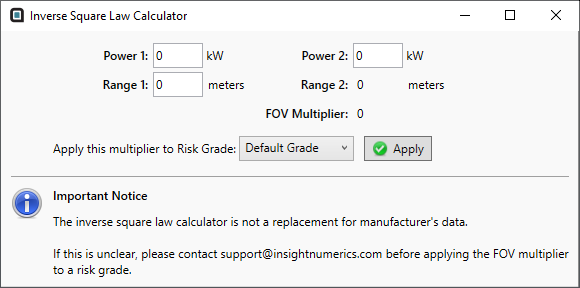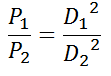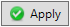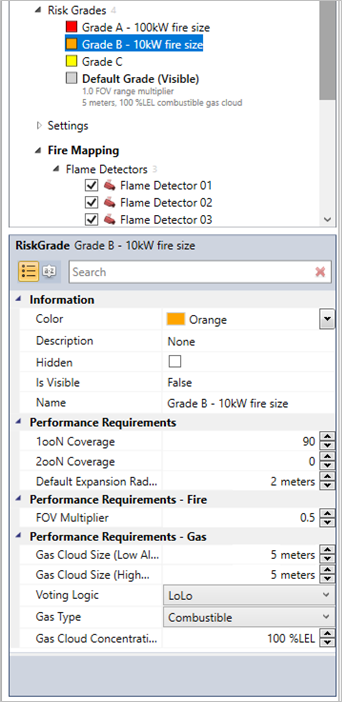# Using Detect3D's Inverse Square Law Tool

Open the Tutorial 4.d3d from the Detect3D Tutorials.zip (22MB) before continuing.

Using the Inverse Square Tool, Detect3D can calculate the corresponding FOV ranges for varying fire powers. Three values are needed as inputs:

• Range 1 - the maximum range of the detector, this is generally the value from the manufacturer

• Power 1 - the RHO value of the fire used when determining the Range 1 distance

• Power 2 - the RHO value of the fire which you want the analysis performed

### What is the power of the 1ft x 1ft pool fire?

The difficulty in using this method is knowing what the Power 1 value should be. In past years, it had been common practice that the 1ft x 1ft n-heptane fire used for testing detectors resulted in a 10kW radiant heat output (RHO). However there is little documentation to support this value of 10kW. In more recent years, with the increased awareness and popularity of F&G mapping, this value has come into question, with some support values closer to 40kW:

• BP’s GP 30-85 (2009 version) references their older standard using a 10kW size but then states “...other sources say this (1ft x 1ft n-heptane fire) should be 40kW” 1 It should also be noted that this note from an older version of BP's GP (pre 2009) mentions use of a 70kW RHO value for the FM3260 test data but does not give any other reference to this value.

• “When using the inverse square law, 37.5kW to 40kW may be used as the basis for the 1ft2 n-heptane fire used to test flame detector FOVs”2,3

However, it is up to the user to determine which value is best for their project for the Power 1 value.

### Inverse Square Law Tool

The below steps will go over opening the Inverse Square Law Tool to determine the FOV Multiplier value needed for a 100kW fire when there is data for a 40kW fire.

1.  From the Project Menu, select theInverse Square Law Tool

2. The below window should appear. Enter a value of "40kW" as Power 1Tutorial 7 - Figure 5 - Inverse Square Law Calculator Window

3. Set the Power 2 value to "100kW". Notice that the FOV Multiplier Value of 1.58 is already calculated and will remain the same regardless of the distance entered in the Range 1 textbox. This is because of the inverse square law relationship where the ratio of the powers (P1 and P2 below) will remain the same regardless of the squared ranges (D1 and D2).Tutorial 7 - Figure 6 - Equation of the relationship between the two power values and two detector distances

4. Enter a value of "30 meters" as the Range 1 and see that the Range 2 value is then calculated as 47.43 meters and doesn't change the FOV Multiplier.

5. The calculated FOV Multiplier can then be applied to an already defined risk grade in the project. Select Grade A and click thebutton to apply the 1.58 value to the FOV Multiplier for Grade A.

6. In the Project Items Tab select Grade A to open the properties panel, and check to ensure that the new value was entered, shown below.Tutorial 7 - Figure 7 - Grade A properties showing the updated FOV multiplier

7. Change the Name of the risk grade to "Grade A - 100kW" and click off the panel to verify the entered text.

Repeat Steps 1 to 7 to calculate the FOV Multiplier for a 10kW fire when the Power 1 value is 40kW. Apply this value to Grade B and change the name accordingly.

Your window should be similar to the below.Continue to the next section to view the updated coverage results.

Footnotes:

1. BP GP30-85 (2009) Appendix C.3

2. SFPE Handbook, section 2

3. Cozzani  V.,  Tugnoli  A.,  Bonvicini  S.,  Salzano  E.,  2013,  9  -Threshold-Based  Approach,  189-207,  2013, Domino Effects in the Process Industries, Eds. Reniers G., Cozzani V., Elsevier, Waltham, MA, USA

4. Mariotti (2014) paper which references Cozzani (2013) https://www.aidic.it/cet/14/36/048.pdf